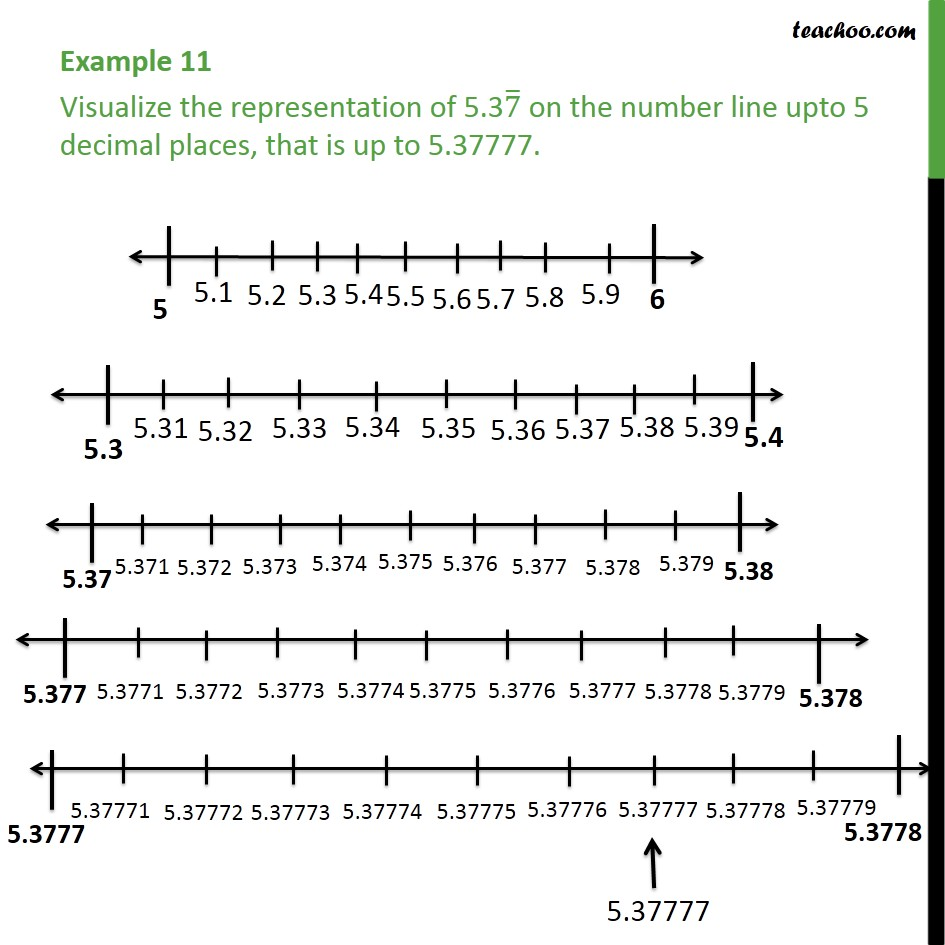1. Chapter 1 Class 9 Number Systems (Term 1)
2. Serial order wise
3. Examples

Transcript

Example 11 Visualize the representation of 5.37 ̅ on the number line upto 5 decimal places, that is up to 5.37777. Here, We need to plot 5.37777 on the number line We follow these steps 1. Since 5.37777 lies between 5 and 6, so making number line between 5 & 6. 2. Now, 5.37777 lies between 5.3 and 5.4, so making number line between 5.3 & 5.4. 3. Similarly, 5.37777 lies between 5.37 and 5.38, so making number line between 5.37 & 5.38. 3. 5.37777 lies between 5.377 and 5.378, so making number line between 5.377 & 5.378. 4. 5.37777 lies between 5.3777 and 5.3778, so making number line between 5.3777 & 5.3778. 5. Marking 5.37777 Let's check the video

Examples